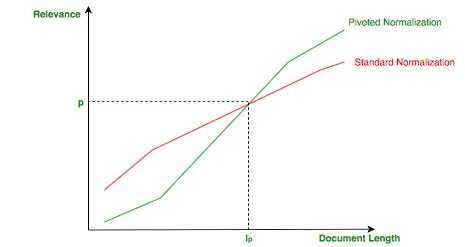Open in App
Not now

# Web Information Retrieval | Vector Space Model

• Difficulty Level : Hard
• Last Updated : 06 Sep, 2021

It goes without saying that in general a search engine responds to a given query with a ranked list of relevant documents.The purpose of this article is to describe a first approach to finding relevant documents with respect to a given query. In the Vector Space Model (VSM), each document or query is a N-dimensional vector where N is the number of distinct terms over all the documents and queries.The i-th index of a vector contains the score of the i-th term for that vector.

The main score functions are based on: Term-Frequency (tf) and Inverse-Document-Frequency(idf).

Term-Frequency and Inverse-Document Frequency –
The Term-Frequency () is computed with respect to the i-th term and j-th document :whereare the occurrences of the i-th term in the j-th document.

The idea is that if a document has multiple receptions of given terms, it will probably deals with that argument.
The Inverse-Document-Frequency () takes into consideration the i-th terms and all the documents in the collection :The intuition is that rare terms are more important that common ones : if a term is present only in a document it can mean that term characterizes that document.
The final scorefor the i-th term in the j-th document consists of a simple multiplication :. Since a document/query contains only a subset of all the distinct terms in the collection, the term frequency can be zero for a big number of terms : this means a sparse vector representation is needed to optimize the space requirements.

Cosine Similarity –
In order to compute the similarity between two vectors : a, b (document/query but also document/document), the cosine similarity is used :

(1)This formula computes the cosine of the angle described by the two normalized vectors : if the vectors are close, the angle is small and the relevance is high.
It can be shown the cosine similarity is the same of the Euclidean distance under the assumption of vector normalization.

Improvements –
There is a subtle problem with the vector normalization: short document that talks about a single topic can be favored at the expenses of long document that deals with more topics because the normalization does not take into consideration the length of a document.

The idea of pivoted normalization is to make document shorter than an empirical value ( pivoted length :) less relevant and document longer more relevant as shown in the following image: Pivoted NormalizationA big issue that it is not taken into consideration in the VSM are the synonyms : there is no semantic relatedness between terms since it is not captured neither by the term frequency nor the inverse document frequency. In order to solve this problems the Generalized Vector Space Model(GVSM) has been introduced.

My Personal Notes arrow_drop_up